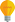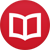# Integral of $\tan(x)$

by Jakub MarianTip: See my list of theMost Common Mistakes in English. It will teach you how to avoid mis­takes with com­mas, pre­pos­i­tions, ir­reg­u­lar verbs, and much more.

The easiest way to integrate $\tan(x)$ is to recall that $$\tan(x) = \frac{\sin(x)}{\cos(x)}\,,$$ so $$∫ \tan(x)\,dx = ∫ \frac{\sin(x)}{\cos(x)}\,dx\,.$$ Do you see the necessary substitution? By choosing $u = \cos(x)$, that is, “$du = -\sin(x)\,dx$” (in quotation marks because this expression does not make sense mathematically, but it does work formally), we get $$∫ \frac{\sin(x)}{\cos(x)}\,dx = ∫ -\frac{1}{u}\,du = -\log(u)+c = -\log(\cos(x))+c$$

By the way, I have written several educational ebooks. If you get a copy, you can learn new things and support this website at the same time—why don’t you check them out?